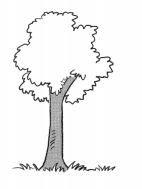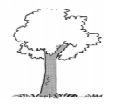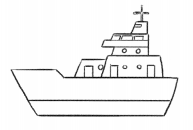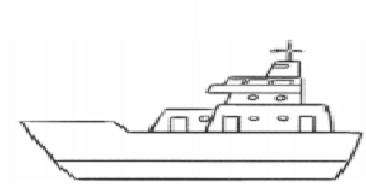# Math in Focus Grade 1 Chapter 9 Practice 1 Answer Key Comparing Two Things

Practice the problems of Math in Focus Grade 1 Workbook Answer Key Chapter 9 Practice 1 Comparing Two Things to score better marks in the exam.

## Math in Focus Grade 1 Chapter 9 Practice 1 Answer Key Comparing Two Things

Example
Which is longer?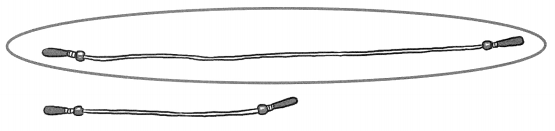Question 1.
Who is taller?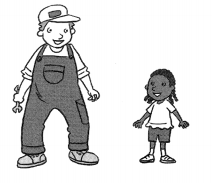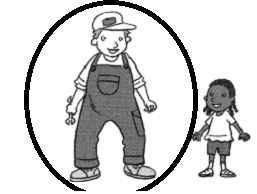Question 2.
Which is shorter?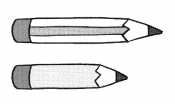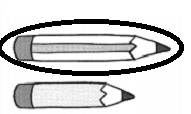Fill in the blanks.

Example

Which is longer? Which is shorter?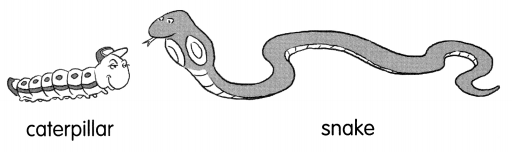The snake is longer than the caterpillar.
The caterpillar is shorter than the snake.

Question 3.
Which is shorter? Which is taller?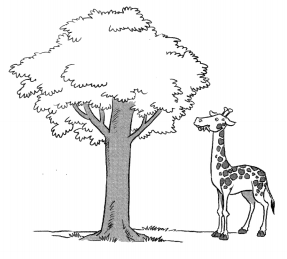The giraffe is ___ than the tree.
The tree is ____ than the giraffe.
The giraffe is shorter than the tree.
The tree is taller than the giraffe.

Question 4.
Which is longer? Which is shorter.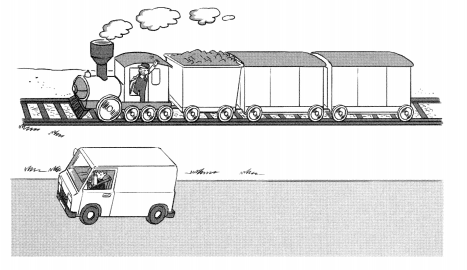The train is ____ than the truck.
The truck is ___ than the train.
The train is longer than the truck.
The truck is shorter than the train.

Question 5.
Which is shorter? Which is taller?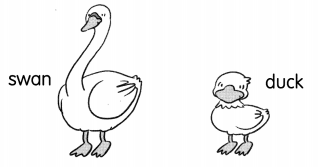The duck is ____ than the swan.
The swan is ___ than the duck.
The duck is shorter than the swan.
The swan is longer than the duck.

Draw.
a longer arrow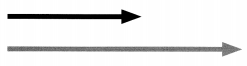Question 6.
a shorter tree